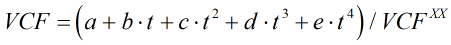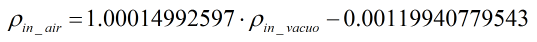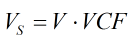# C301 – ASTM D1555 – Volume and Weight of Industrial Aromatics and Cyclohexane

## Description

This calculates the volume at standard conditions and weight of cyclohexane and aromatic compounds commonly used for industrial application. It calculates weight and density on a in vacuo and in air basis.

ASTM D1555-09 – Standard Test Method for Calculation of Volume and Weight of Industrial Aromatic Hydrocarbons and Cyclohexane (2009)

ASTM D1555M-08e1 – Standard Test Method for Calculation of Volume and Weight of Industrial Aromatic Hydrocarbons and Cyclohexane [Metric] (2009)

Kelton calculation reference C301

FLOCALC calculation reference F101

KIMS calculation reference K236

## Options

### Product

• Benzene
• Cumene
• Cyclohexane
• Ethylbenzene
• Styrene
• Toluene
• m-Xylene
• o-Xylene
• p-Xylene
• Mixed Xylenes
• 300-350°F Aromatics
• 350-400°F Aromatics

This option is used to select the product being measured.

### Standard Temperature

• 15°C
• 20°C
• 60°F

This option is used to select the temperature which the volume is being corrected to. If 60°F is selected the procedure used is that laid out in ASTM D1555-09, otherwise it follows the procedure in ASTM D1555M-08e1.

### Purity

• Impure products

This option is checked if the product being measured is less than 100% pure. In which case density is obtained by measurement and will now appear on the inputs tab.

### Rounding

• Apply

This option is selected to apply rounding to 5 significant figures to values with in the calculation. If this option is not selected then calculations will be done to full (double) precision.

## Calculation

### Volume Correction Factor

The volume correction factor, VCF, is calculated by:Where a = Constant from Table 3 b = Constant from Table 3 c = Constant from Table 3 d = Constant from Table 3 e = Constant from Table 3 VCFXX = Constant from Table 3 (if 60°F is selected as standard temperature this will be set to 1)

### Density in air

The density in air is calculated by:Where ρin_vacuo = density in vacuo

### Volume

The volume at the standard temperature is calculated by:Where V = Volume at ambient temperature

### Weight

Weight is calculated from:Where Vs = Volume at standard temperature ρ = density

Note that if the density used is the density in vacuo this equation will give weight in vacuo and if the density in air is used it will give weight in air.

Back